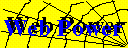# Power Analysis Help

### Specifying Effect Size

Determining the sample size for a factor or effect in an ANOVA design is usually difficult because of the need to specify all of the treatment means in order to calculate the non-centrality parameter of the F-distribution, on which power depends.

Effect size (delta) is specified by the difference between the largest mean and the smallest mean, in units of the within-cell standard deviation ( sigma = the square root of the MSE):

```            largest mean - smallest mean
delta   =   ----------------------------
sigma
```
The minimum power specification corresponds to the alternative hypothesis that all means other than the two extreme one are equal to the grand mean. The computations assume: (a) fixed effects, and (b) equal sample sizes in all treatments. Under these assumptions, the non-centrality parameter of the F-distribution can be calculated as N (delta^2)/2, where N is the sample size per treatment.

Effect size delta values are typically in the range of 0 - 3. In social science applications, values of delta = 0.25, 0.75, and 1.25 or greater correspond to "small", "medium", and "large" effects, according to Cohen & Cohen, Statistical Power Analysis for the Behavioral Sciences.

### Factorial Designs

The program was designed to calculate power for a main effect in one-way and two-way factorial designs with fixed effects.

However, the program can also be used for ANY fixed effect in ANY crossed factorial design, by designating the levels of the effect of interest as 'A', and the levels of all other crossed factors as 'B'.

Examples:

• Specify b=1 for a one-way design.
• In a 4 x 3 x 2 design, there are 6 combinations of factors 2 and 3 for each level of the first factor, so specify b=6. The sample sizes calculated are for each level of Factor A, so divide the reported values by 6 to get treatment cell sizes.
• To determine the power or sample size for the BxC interaction in a 4x3x2 design, specify a=6 (the combinations of factors B and C), and b=4 (levels of factor A for each BC combination. The delta values here refer to the BC treatment means.

Michael Friendly
Note:This application is provided as free service. I am unable to answer e-mail concerning power analysis.